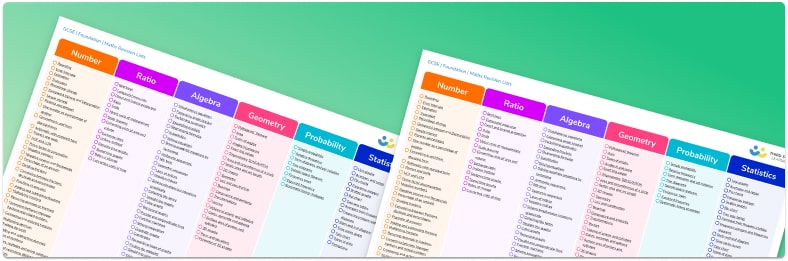# GCSE Maths Topic ListA GCSE maths topic list for every student to focus revision for their exams

• Separate single page maths checklists for foundation tier and higher tier
• Each topic links to the Third Space Learning GCSE maths revision exam guides where you will find step by step examples, practice questions and exam questions.
• The maths topic lists also provide quick links to more free downloadable resources including worksheets, diagnostic questions, revision mats and much more!
• Lists include: 90+ algebra topics, 95+ number topics, 150+ geometry topics, 45+ statistics topics, 40+ ratio and proportion topics, 25+ probability topics

• This field is for validation purposes and should be left unchanged.

You can unsubscribe at any time (each email we send will contain an easy way to unsubscribe). To find out more about how we use your data, see our privacy policy.

90+ Algebra topics including:

• inequalities
• formulae
• factorising
• graphs
• indices
• algebraic fractions
• cubic graphs
• nth term
• simultaneous equations
• exponentials
• linear equations
• simplifying
• pythagoras’ theorem
• straight line graphs

95+ Number topics including:

• decimals
• percentages
• fractions
• factors and multiples
• standard form
• hcf and lcm
• place value
• surds
• number lines
• percentage change

150+ Geometry topics including:

• vectors
• constructions
• polygons
• trigonometry
• circle theorems
• sine rule
• cosine rule
• enlargements
• similar shapes
• surface area
• congruent shapes
• loci
• negative scale factors
• parallel lines

45+ Statistics topics including:

• box plots
• cumulative frequency
• pie charts
• scatter graphs
• averages
• histograms
• two way tables
• real life graphs

40+ Ratio and Proportion topics including:

• rates of change
• proportion and inverse proportion
• conversions

25+ Probability topics including:

• tree diagrams
• venn diagrams
• frequency trees

## Do you have KS4 students who need more focused attention to succeed at GCSE?There will be students in your class who require individual attention to help them succeed in their maths GCSEs. In a class of 30, it’s not always easy to provide.

Help your students feel confident with exam-style questions and the strategies they’ll need to answer them correctly with our dedicated GCSE maths revision programme.

Lessons are selected to provide support where each student needs it most, and specially-trained GCSE maths tutors adapt the pitch and pace of each lesson. This ensures a personalised revision programme that raises grades and boosts confidence.

Find out more